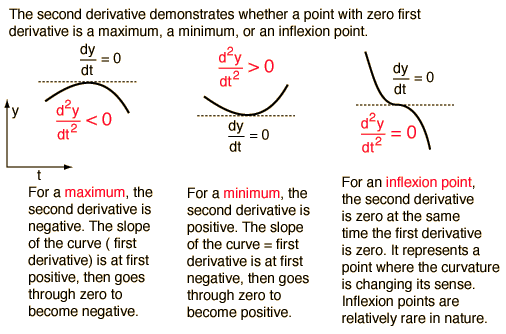# Training and learning

Caution! This article is 3 years old. It may be obsolete or show old techniques. It may also still be relevant, and you may find it useful! So it has been marked as deprecated, just in case.

## Training

When we train a neural network, we are trying to optimize the weights associated with each input in each layer of the model, so that they accurately map the input data to the correct output class (each node of the output layer is a different class).

The weights are optimized using what we call an "optimization algorithm". There are several, but one of the most used is the Stochastic Gradient Descent or SGD. The objective of this function is to find the best set of weights that minimize the loss function.

The results will depend on both the optimizer and loss function we choose to use when training our model.

The output produced by the neural network is a set of values of probability for every class we have. For example, if we want the network to tell us if a picture is a cat or a dog, we will have two classes at the end: cat and dog. So if we pass it a picture of a cat, the output could be 75% probability that it is a cat and 25% probability that it is a dog.

• The loss is the error or difference between what the network is predicting for the image versus the true label of the image, and SGD will to try to minimize this error to make our model as accurate as possible in its predictions.

• Training is passing the same data over and over again to the neural network. During this process, the model learns from the data. An epoch refers to each single pass of the entire dataset to the network during training.

## Learning

The training starts with arbitrary values for the network weights and the loss is calculated at the end of the first epoch by comparing prediction with reality. Then we do a backpropagation: we calculate the gradient of this loss with respect to each of the weights. In other words, the gradient is the derivative of the loss function (L) with respect to each weight (w):

``````
L = f(w1, w2, ...wN)

gradient = dL / dw
= ∂L / ∂w1 + ∂L / ∂w2 + ... + ∂L / ∂wN
``````

Once we have the value for the gradient of the loss function, we can use this value to update the model’s weights. The gradient tells us which direction will move the loss towards the minimum. The loss function has a minimum where the first derivative is zero and the second derivative is positive.Explanation on how to find maximum, minimum and inflection point of a function, using its first and second derivatives.

We then multiply the gradient value by something called a learning rate. A learning rate is a small number usually ranging between 0.01 and 0.0001. The learning rate tells us how large of a step we should take in the direction of the minimum, and it can be different per layer.

``````
new weight = old weight - (learning rate * gradient)
``````

This updating of the weights is what "learning" means.

An example of how we would train such a model in Keras, using a variant of the SDG optimizer called Adam (read the paper here) and a network with two hidden dense layers, can be found below:

``````
import numpy
from tensorflow.keras.models import Sequential
from tensorflow.keras.layers import Dense
from tensorflow.keras.optimizers import Adam

BATCH_SIZE = 3
train_samples = numpy.array([
[150, 67], [130, 60], [200, 65], [125, 52], [230, 72], [181, 70]
])
train_labels = numpy.array([1, 1, 0, 1, 0, 0])

model = Sequential([
Dense(units=8, input_shape=(1,), activation='relu'),
Dense(units=16, activation='relu'),
Dense(units=2, activation='sigmoid')
])
model.summary()

model.compile(